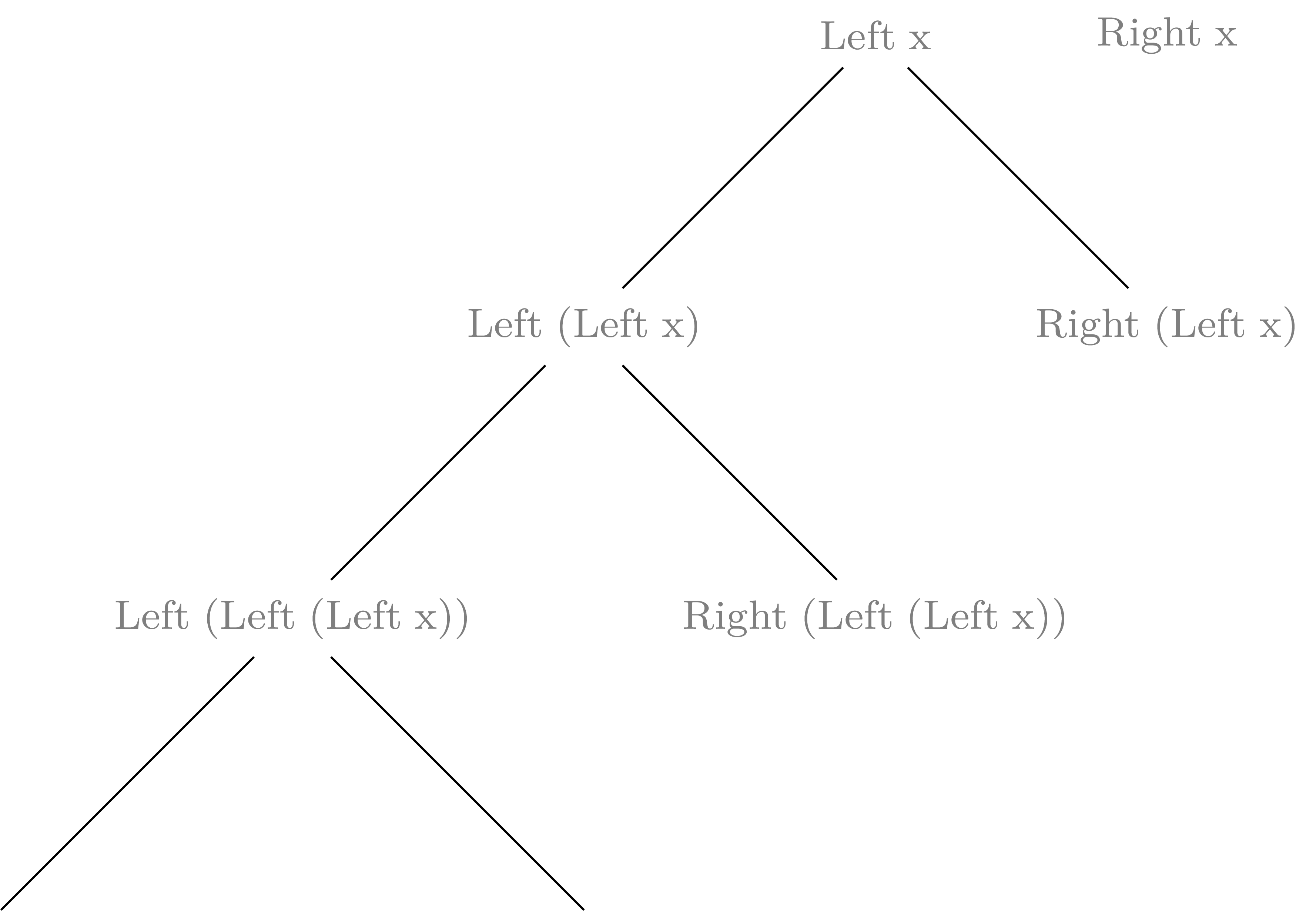Universal algebra is a field of mathematics which deals with general algebraic structures. Much of classical algebra is about groups (e.g. integers with addition, or rotations of a plane), rings (e.g. the ring of integers with addition and multiplication), fields (e.g. real numbers, or complex numbers, where one multiplicative inverses of non 0 elements exists). Universal algebra deals with more general structures like that. Every algebra has the underlying universum - a set of numbers and a set of operations. The operations can have different arity, i.e. number of arguments. Examples include constants - operations of arity 0, or multiplication, addition of integers - arity 2, or inverse - arity 1.

## Peano algebras

Let’s take as an example the algebra of natural numbers using Peano axioms. It has one constant - let’s call it $0$ and one operation of arity 1 - the successor function $s$. In Universal Algebra one can consider all of the algebras which has this set of operations and satisfies the Peano axioms, but let us skip the following two:

• the injectivity axiom of $s$:

$$s(m) = s(n) \Rightarrow m = n$$
• 0 is not a successor of any element:

$$\not\exists_n s(n) = 0$$

Otherwise there would be only one algebra to consider - natural numbers – at the end Peano was looking for axiomatic formulation of natural numbers, hence the two requirements.

Let us call algebras which satisfy these axioms Peano algebras. One example is the set of natural numbers $\mathbb{N}$, where the successor is defined as $s(n) = n + 1$. This is a free algebra with one generator. What it really means is that all elements can be generated from $0$ and the operation $s$, and that there is no relation/equations between elements. A non free example would be the set of $\mathbb{N}/2\mathbb{N} \simeq \{0,1\}$, where $s(0) = 1$ and $s(1) = 0$ (this breaks the two original Peano axioms that that we excluded).

It turns out that Peano algebras are often met in Haskell code. Let’s take Haskell's Maybe type. It has two constructors Nothing and Just, where Nothing has kind $*$ (arity 0) and Just has kind $*\rightarrow *$ (arity 1); like $0$ and $s$ in the Peano algebra case. And indeed it forms a Peano Algebra, for any type $a$. It’s elements are:

Unit, Maybe Unit, Maybe (Maybe Unit), ...

This algebra is free, there are no relations (or equalities of types) among all algebras of this type. But relations are very useful, and it turns out that Haskell provides them using monads. For example for the Maybe type we have

(Just (Just x) >>= id) == Just x

for every x :: a. Note that this equation is on the level of terms rather than types.

This way when you use Maybe in a monadic context you use it as $\mathbb{N}/2\mathbb{N}$ rather than the free algebra $\mathbb{N}$. Knowing these relations is the essence of knowing how a given monad works.

There are other examples of a Peano algebras in Haskell. The Either monad provides an example with two generators - or two data constructors Left and Right. As a Peano algebra it can be represented as a binary tree where the successor of Right x is Right (Right x) and the successor of Left x is Left (Left x) (for some type x). Then the Either monad provides only one relation:

(Right (Right x) >>= id) == Right x

and there is no relation for the Left constructor:

(Left (x :: Either a) >>= id) == Left x

for every term x :: a. The corresponding Peano algebra has the form: